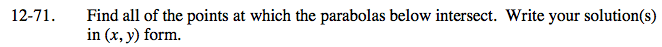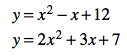### Home > CAAC > Chapter 12 > Lesson 12.4.1 > Problem12-71

12-71.Set the equations equal to each other.

x² - x + 12 = 2x² + 3x + 7

Set the equation equal to zero.

0 = x² + 4x − 5

Factor the expresion.

Solve for x.